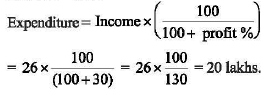# UGC NET Paper 1 December (Pack of 7) - UGC NET Paper 1 5th December 2019 Evening Shift

>>>>>>>>UGC NET Paper 1 5th December 2019 Evening Shift

Directions: The table below presents the percentage profit earned by two companies A and B during the period 2013 to 2018. Based on the data given in the table, answer five questions that follow

Yearwise percentage (%) profit

 Year % Profit Earned A B 2013 20% 35% 2014 32% 30% 2015 40% 50% 2016 25% 40% 2017 30% 30% 2018 15% 40%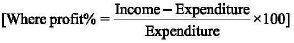• A

2013• B

2017• C

2015• D

2018• Option : C
• Explanation :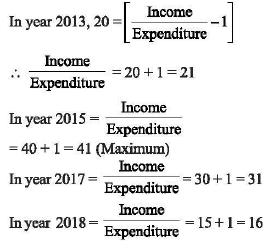Hence, the ratio is maximum in year 2015.

Directions: The table below presents the percentage profit earned by two companies A and B during the period 2013 to 2018. Based on the data given in the table, answer five questions that follow

Yearwise percentage (%) profit

 Year % Profit Earned A B 2013 20% 35% 2014 32% 30% 2015 40% 50% 2016 25% 40% 2017 30% 30% 2018 15% 40%• A

62.4 Lakhs• B

36.2 Lakhs• C

64.0 Lakhs• D

65.5 Lakhs• Option : A
• Explanation :
Sum of expenditure of company B in year 2014 and 2017 = 481akhs.
Percent of profit of company B in year 2014 and 2017 is 30% each
Let expenditure of company B in year 2014 and 2017 are x and (48 - x) respectively
So, Income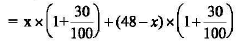= 1.3x + (48-x) x 1.3
= 1.3 x (48)
= 1.3 x 48 ≈ 62.4 lakhs.

Directions: The table below presents the percentage profit earned by two companies A and B during the period 2013 to 2018. Based on the data given in the table, answer five questions that follow

Yearwise percentage (%) profit

 Year % Profit Earned A B 2013 20% 35% 2014 32% 30% 2015 40% 50% 2016 25% 40% 2017 30% 30% 2018 15% 40%• A

22.95 Lakhs• B

23.15 Lakhs• C

24.50 Lakhs• D

25.65 Lakhs• Option : A
• Explanation :
Expenditure of company B in year 2013 = 171akhs
Profit% = 35%Directions: The table below presents the percentage profit earned by two companies A and B during the period 2013 to 2018. Based on the data given in the table, answer five questions that follow

Yearwise percentage (%) profit

 Year % Profit Earned A B 2013 20% 35% 2014 32% 30% 2015 40% 50% 2016 25% 40% 2017 30% 30% 2018 15% 40%• A

55 Lakhs• B

66 Lakhs• C

56 Lakhs• D

64 Lakhs• Option : B
• Explanation :
Income of company Bin 2018 = 77 lakhs
Profit % = 40%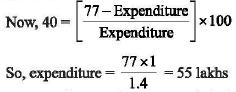∴ Expenditure of company A in 2013 = 55lakhs
Now, profit % = 20%
∴ Income of company A in 2013
= 55 x {1 + (20/100)}
= 55 x 1.2 = 66 lakhs

Directions: The table below presents the percentage profit earned by two companies A and B during the period 2013 to 2018. Based on the data given in the table, answer five questions that follow

Yearwise percentage (%) profit

 Year % Profit Earned A B 2013 20% 35% 2014 32% 30% 2015 40% 50% 2016 25% 40% 2017 30% 30% 2018 15% 40%• A

33.8 Lakhs• B

22.5 Lakhs• C

21.6 Lakhs• D

20.0 Lakhs• Option : D
• Explanation :
Income of company A in 2017 = 26 lakhs
Profit % = 30%